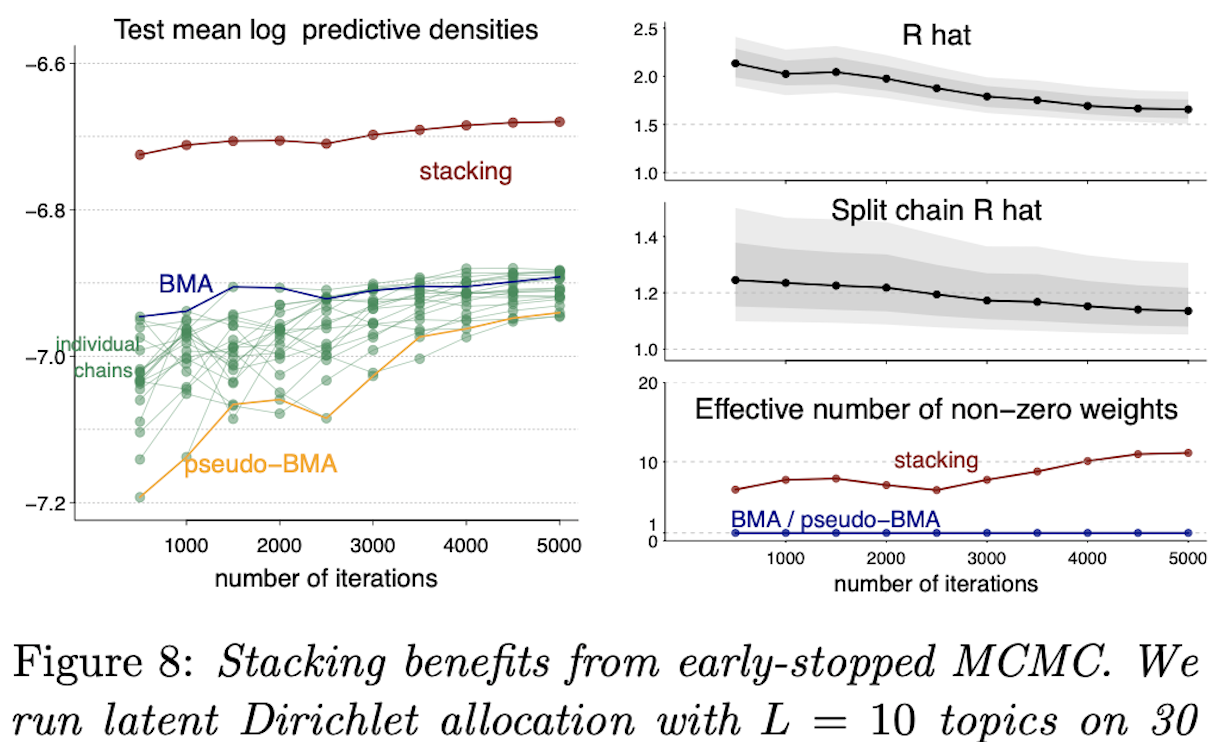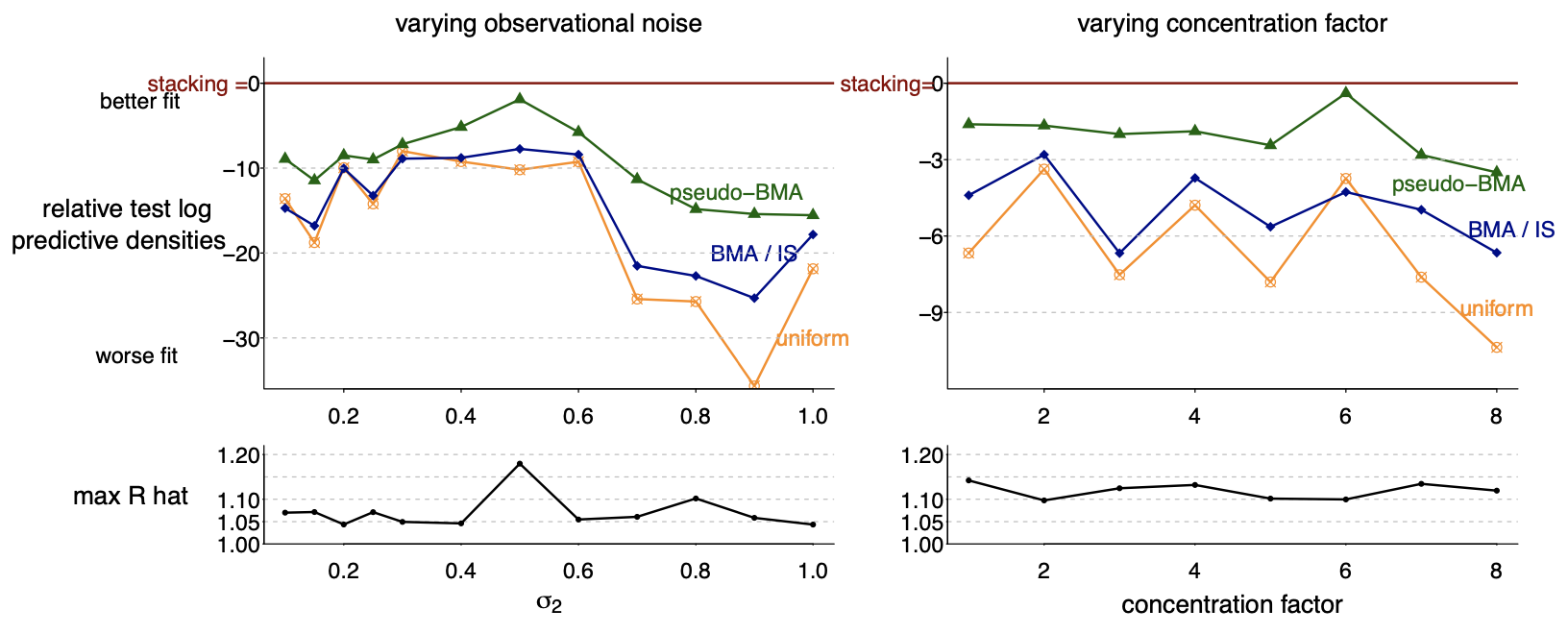# Stacking for Non-mixing Bayesian Computations: The Curse and Blessing of Multimodal PosteriorsWhen working with multimodal Bayesian posterior distributions, Markov chain Monte Carlo (MCMC) algorithms can have difficulty moving between modes, and default variational or mode-based approximate inferences will understate posterior uncertainty. And, even if the most important modes can be found, it is difficult to evaluate their relative weights in the posterior.

Here we propose an alternative approach, using parallel runs of MCMC, variational, or mode-based inference to hit as many modes or separated regions as possible, and then combining these using importance sampling based Bayesian stacking, a scalable method for constructing a weighted average of distributions so as to maximize cross-validated prediction utility. The result from stacking is not necessarily equivalent, even asymptotically, to fully Bayesian inference, but it serves many of the same goals. Under misspecified models, stacking can give better predictive performance than full Bayesian inference, hence the multimodality can be considered a blessing rather than a curse.

We explore with an example where the stacked inference approximates the true data generating process from the misspecified model, an example of inconsistent inference, and non-mixing samplers. We elaborate the practical implantation in the context of latent Dirichlet allocation, Gaussian process regression, hierarchical model, variational inference in horseshoe regression, and neural networks.

Poor mixing of MCMC (and other algorithms such as variational inference) is inevitable, either because of fundamental discreteness (multimodality) in the posterior distribution, or diverse geometry arising from the desire to fit a model that represents a multitude of explanations for data, or just because you want to work fast and in parallel. What, then, to do with all these snips from the posterior distribution? It turns out that Bayesian model averaging (giving each snip a weight corresponding to its estimated mass in the posterior distribution) doesn’t always work so well, in part for the same mathematical reasons that Bayes factors don’t work in an M-open world. We find that cross-validated model averaging (Bayesian stacking) works better.

Stacking of parallel chains can even be superefficient, outperforming full Bayes because it can catch model failures, in a way similar to the mixture model formulation of Kamary, Mengersen, Robert, and Rousseau. And you can flip the idea around and use the stacking average to check model fit.

We can implement stacking in Stan by computing the vector of the log posterior density in the generated quantities block, and then using Pareto smoothed importance sampling to compute leave-one-out cross validation without having to re-fit the model n times.

I think this is a big idea, both for throwing at difficult problems in Bayesian computation and for facilitating a faster workflow using parallel simulation.